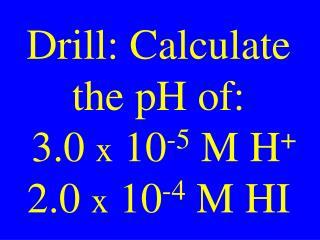DownloadDownload PresentationDrill: Calculate the pH of: 3.0 x 10 -5 M H + 2.0 x 10 -4 M HI

# Drill: Calculate the pH of: 3.0 x 10 -5 M H + 2.0 x 10 -4 M HI

Download Presentation## Drill: Calculate the pH of: 3.0 x 10 -5 M H + 2.0 x 10 -4 M HI

- - - - - - - - - - - - - - - - - - - - - - - - - - - E N D - - - - - - - - - - - - - - - - - - - - - - - - - - -
##### Presentation Transcript

1. Drill: Calculate the pH of: 3.0 x 10-5 M H+ 2.0 x 10-4 M HI

2. Review Drill&Check HW

3. CHM II HW • Review PP-22 • Complete the attached assignment & turn it in tomorrow.

4. Are there any questions on previous material?

5. Get Data From The Standard Solution Lab

6. Acid-Base Equilibria

7. Ionization of Water H2O(l) H+(aq)+ OH-(aq) 2 H2O(l) H3O+(aq) + OH-(aq)

8. Water Ionization Constant Kw = [H+][OH-] or Kw = [H3O+][OH-] Kw = 1.00 x 10-14 Memorize this

9. Kw Calculations In pure water [H+] = [OH-] Kw =[H+][OH-] = 1.0 x 10-14 Let [H+] = x = [OH-]

10. Kw Calculations Kw =[H+][OH-] = x2 Kw = x2 =1.0 x 10-14 Thus x = 1.0 x 10-7 M [H+] = x = 1.0 x 10-7 M

11. pH of Pure Water [H+] = 1.0 x 10-7 M pH = -log[H+] pH = -log[1.0 x 10-7] pH = 7 -log 1 Thus pH = 7.00

12. Calculate the pH of 3.3 x 10-8 M HI

13. Calculate [H+],[OH-], pH, & pOH of 0.020 M HCl

14. Calculate [H+] of 0.050 M H2SO4

15. Weak Acid Ionization HA(aq) H+(aq) + A-(aq) HA(aq) + H2O(l) H3O+(aq) + A-(aq)

16. Acid Dissociation Constant HA(aq)H+(aq) + A-(aq) • [H+][A-] • [HA] Ka =

17. Weak Base Ionization NH3(aq) + H2O(l) NH4+(aq) + OH-(aq)

18. Base Dissociation Constant NH3(aq) + H2O(l) NH4+(aq) + OH-(aq) • [NH4+][OH-] • [NH3] Kb =

19. Drill: Calculate [H+],[OH-], pH, & pOH of 0.025 M KOH

20. Review & Collect Drill & HW

21. CHM II HW • Review PP-22 • Complete the attached assignment & turn it in tomorrow.

22. Make sure you copy the Std KHP molarities

23. Are there any questions on previous material?

24. Acid-Base Equilibria Problems

25. Calculate [H+], [OH-], pH, & pOH of0.50 M NH3Kb = 1.8 x 10-5

26. Drill: Calculate [H+] & pH of 0.50 M C6H5NH2Kb = 3.2 x 10-5

27. Drill: Calculate [H+] & pH of 0.50 M NaOH

28. Review Drill&Check HW

29. Collect Lab

30. CHM II HW • Review PP-22 • Complete the attached assignment & turn it in tomorrow.

31. Are there any questions on previous material?

32. Calculate the Ka of 0.10 M Hquack when it ionized 5.0 % in an aqueous solution.

33. Drill: Calculate the pH of0.025 M HF:Ka = 6.4 x 10-4

34. Drill: Calculatethe pH of 0.025 M QOH:Kb = 2.0 x 10-4

35. You have 10 identical coins with 1 coin having a slight mass difference. Determine the odd coin with only 3 uses of an equal arm balance.

36. Drill: Calculate the pH of 0.25 M HAz Ka = 2.5 x 10-3

37. Review Drill&Check HW

38. CHM II HW • Review PP-22 • Complete the attached problem & turn it in tomorrow.

39. CHM II Schedule • Lab Tomorrow • Review on Wednesday • Test on Thursday • Labs attached

40. Are there any questions on previous material?

41. PolyproticAcidEquilibria

42. Calculate [H2CO3], [HCO3-], [CO3-2], [H+],[OH-], & pH of 0.44 M H2CO3Ka1 = 4.4 x 10-7Ka2 = 4.7 x 10-11

43. Drill: Calculate the pH of 0.25 M HAz Ka = 2.5 x 10-3

44. Review & Collect Drill

45. Schedule • Lab: tomorrow • Lab Wednesday • Review Thursday

46. CHM II Test covering A/B & A/B Equilibria will be on Friday